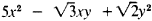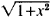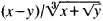# Algebraic Function

Also found in: Dictionary, Wikipedia.

## algebraic function

[¦al·jə¦brā·ik ′fəŋk·shən]
(mathematics)
A function whose value is obtained by performing only the following operations to its argument: addition, subtraction, multiplication, division, raising to a rational power.
McGraw-Hill Dictionary of Scientific & Technical Terms, 6E, Copyright © 2003 by The McGraw-Hill Companies, Inc.
The following article is from The Great Soviet Encyclopedia (1979). It might be outdated or ideologically biased.

## Algebraic Function

a function that satisfies an algebraic equation; one of the most important functions studied in mathematics. Among the algebraic functions, polynomials and quotient polynomials—for example,and (1 + x + x2)/(2 + x3)—are called rational and all the rest are called irrational. The simplest examples of the latter are algebraic functions expressed by means of radicals—for example,andHowever, there are certain algebraic functions that cannot be expressed with radicals—the function y = f (x) which satisfies the equation y5 + 5yx4 + 5x5 = 0. Examples of nonalgebraic, or so-called transcendental functions, encountered in school algebra courses, are the power function xα, if α is an irrational number; the exponential function ax; the logarithmic function; and so forth. The general theory of algebraic functions is an extensive mathematical discipline having important connections with the theory of analytic functions (of which algebraic functions constitute a special class), algebra, and algebraic geometry. The most general algebraic function of many variables, u = f(x, y, z., ...), is defined as a function satisfying an equation of the type

P0(x, y, z, . . . )un + P1 (x, y, z, . . .)un−1

(1) + . . . + Pn (x, y, z, . . . ) = 0

where P0,P1,. . . Pn are any polynomials with respect to x, y, z, . . . . The entire expression in the left member represents a certain polynomial with respect to x, y, z, . . . and u. It may be considered irreducible—that is, not factorable into polynomials of lower degree; in addition, polynomial P0 can be considered as not identically equal to zero. If n = 1, then u represents a rational function (u = −P1/P0), a partial case of which—the integral rational function—is the polynomial (if P0 = const ≠ 0). When n > 1, we have an irrational function; when n = 2, the function is expressed in terms of polynomials with the use of a square root; when n = 3 or 4, for u we have an expression containing both square and cube roots.

When n ≥ 5, the irrational function u can no longer be expressed in the general case, in terms of a finite number of any roots of polynomials. An irrational algebraic function is always many-valued; it is, precisely, for our given designations and assumptions, an n-valued analytic function of variables x, y, z,.....

### REFERENCE

Chebotarev, N. G. Teoriia algebraicheskikh funktsii. Moscow-Leningrad, 1948.
The Great Soviet Encyclopedia, 3rd Edition (1970-1979). © 2010 The Gale Group, Inc. All rights reserved.
References in periodicals archive ?
The relation between the algebraic function S from (3.2) is given by
The proposed nonlinear system is designed using a linear part added to a nonlinear algebraic function, leading to the structure:
The method of algebraic function approximation in eigenvalue problems of closed lossless waveguides has been illustrated by means of two examples .
Both Mathematica 6.0.2 and the TI-89 did successfully integrate the algebraic function of "u" described above; however, the TI-89 gave no result for the original integral, and Mathematica 6.0.2 gave a solution using complex elliptic F and Pi functions.
Algebraic numbers and algebraic functions. (reprint, 1967)
4 Asymptotics for coefficients of algebraic function
Let then the algebraic function y = y (z) be defined by
Applicat ion of the Cagniard-de Hoop method requires a detailed discussion of the structure of a moderately complicated algebraic function accompanying the transform of the integration path.
Each element, or more properly, cell, is described as a high-level algebraic function. In fact, the real efficiency of BEM was lost if an engineer attempted to "overmesh" by applying a FEM meshing strategy.
of Tokyo) explore similarities between algebraic number fields and algebraic function fields in one variable over finite fields, explain adele rings and idele groups, derive several prime number theorems, and prove the main theorem of class field theory.
Since F is rational, these singularities are poles and algebraic functions of x, so that the residue sum, the diagonal generating function, is also an algebraic function of x.

Site: Follow: Share:
Open / Close